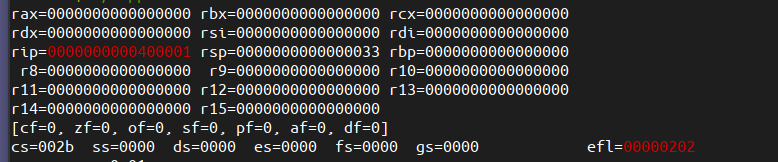Rappel is a wonderful for understanding the basics of assembly language. Initially assembly language is quite challenging to understand for the programmer as all these registers are quite confusing. Specially it becomes more difficult because the programmer is now limited to using only handful of variables where as in other languages, the number declaring variable appears to be unlimited.

``````\$ ./rappel
rax=0000000000000000 rbx=0000000000000000 rcx=0000000000000000
rdx=0000000000000000 rsi=0000000000000000 rdi=0000000000000000
rip=0000000000400001 rsp=0000000000000033 rbp=0000000000000000
r8=0000000000000000  r9=0000000000000000 r10=0000000000000000
r11=0000000000000000 r12=0000000000000000 r13=0000000000000000
r14=0000000000000000 r15=0000000000000000
[cf=0, zf=0, of=0, sf=0, pf=0, af=0, df=0]
cs=002b  ss=0000  ds=0000  es=0000  fs=0000  gs=0000            efl=00000202
``````

The below is a screenshot of rappel.